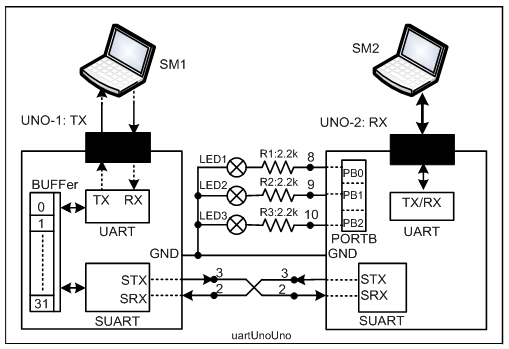# Serial communication transfer data btw 2 arduino

hi.
i want to transfer 3 data from master to slave and turn on / off led in slave.
HOW COULD I TRANSFER 3 DATA IN SERIAL COMMUNICATION BTW 2 ARDUINO UNO?
if data1 = 0 in master , led 1 = LOW in slave, if data1 = 1 , led1 in master = High in slave and like this for two other one.
how could i do this?

code in master:

``````int a1 = 0;
int a2 = 0;
int a3 = 0;
void setup() {
Serial.begin(9600);
pinMode (1,INPUT); // PIN 1 CONNECTED TO A SWITCH = 0 OR 1
pinMode (2,INPUT); // PIN 2 CONNECTED TO A SWITCH = 0 OR 1
pinMode (3,INPUT); // PIN 3 CONNECTED TO A SWITCH = 0 OR 1
}

void loop() {

/// .... i used Serial.write(a1) and erial.write(a2) and erial.write(a3) but it didn't transfer correct from master to slave. how could i write next code:

}
``````

Code in Slave:

``````// slave code:
// This code can't get data correctly from master.
int a1;
int a2;
int a3;

void setup() {
Serial.begin(9600);
pinMode (3,OUTPUT);
pinMode (4,OUTPUT);
pinMode (5,OUTPUT);
}

void loop() {

delay (500);
delay (500);

if (a1 == 1)
{
digitalWrite (3,HIGH);
}
if (a2 == 1)
{
digitalWrite (4,HIGH);
}
if (a3 == 1)
{
digitalWrite (5,HIGH);
}
if (a1 == 0)
{
digitalWrite (3,LOW);
}
if (a2 == 0)
{
digitalWrite (4,LOW);
}
if (a3 == 0)
{
digitalWrite (5,LOW);
}
}
``````
``````pinMode (1,INPUT); // PIN 1 CONNECTED TO A SWITCH = 0 OR 1
``````

Don't use pins 0 or 1. They are used by the Serial interface and they can't do 2 things at once

I correct that.
now how i should program this problem?
HOW COULD I TRANSFER 3 DATA IN SERIAL COMMUNICATION BTW 2 ARDUINO UNO?

how do you expect to synchronize transmission, identify the first value?

you might be better off sending 3digits with a newline terminator.

My Arduino to Arduino via Serial tutorial goes into this in detail with both CSV and json examples. json is usually an overkill in my opinion.
That tutorial also have a SerialComs class that works will with SoftwareSerial and is very tolerant of long delays on either side.

1. Build the following hardware setup of Fig-1.Fig-1:

2. Upload the following sketch in UNO-1 (untested)

``````#include<SoftwareSerial.h>
SoftwareSerial SUART(2, 3);  //SRX=DPin-2, STX = DPin-3

void setup()
{
Serial.begin(9600); //UART Port is enabled
SUART.begin(9600);  //SUART Port is enabled
}

void loop()
{
byte n = SUART.available(); //check if a data byte has come from InputBox
if(n == 3)//three bytes have arrived from InputBox For example: 0(MSB)10
{
SUART.print(SUART.read()); 0(LSB) goes to UNO-2 as 0x30 (ASCII Code)
SUART.print(SUART.read()); 1 goes as 0x31 (ASCII Code)goes to UNO-2
SUART.print(SUART.read()); 0(MSB=Most significant Byte) goes to UNO-2
}
}
``````

3. Upload the following sketch in UNO-2 (untested).

``````#include<SoftwareSerial.h>
SoftwareSerial SUART(2, 3);  //SRX=DPin-2, STX = DPin-3

void setup()
{
Serial.begin(9600); //UART Port is enabled
SUART.begin(9600);  //SUART Port is enabled
pinMode(8, OUTPUT);
pinMode(9, OUTPUT);
pinMode(10, OUTPUT);
}

void loop()
{
byte n = SUART.available(); //check if a data byte has come from InputBox
if (n == 3) //three bytes have arrived from InputBox For example: 0(MSB)10
{
digitalWrite(8, SUART.read()); //0(LSB) goes to UNO - 2 as 0x30 (ASCII Code)
digitalWrite(9, SUART.read()); //1 goes as 0x31 (ASCII Code)goes to UNO - 2
digitalWrite(10, SUART.read()); //0(MSB = Most significant Byte) goes to UNO - 2
}
}
``````

4. Open Serial Monitor (Fig-2) of UNO-1 at 9600 Bd. Choose Line ending tab to "No line ending".

Fig-2:
5. Enter 010 in the InputBox of Serial Monitor and then click on the Send button.

6. Check that LED2 of Receiver is ON.

7. Try other 3-bit patter and check that the corresponding LED of Receiver has turned ON.

This topic was automatically closed 120 days after the last reply. New replies are no longer allowed.